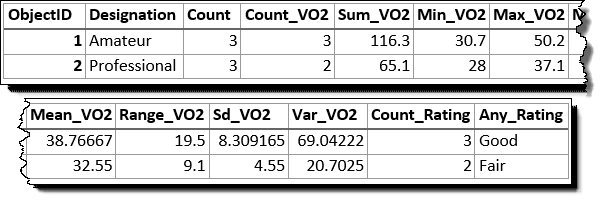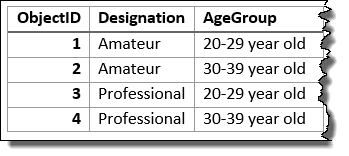# Summarize Attributes (GeoAnalytics Desktop)

## Summary

Calculates summary statistics for fields in a feature class.

## Usage

• Summarize Attributes is a tabular analysis tool, not a spatial analysis tool. Inputs can be a tabular layer or a layer with geometry (points, lines, or polygons).

• You can specify one or more fields to summarize by or summarize all features. When you summarize by fields, statistics are calculated for each unique combination of attribute values.

• The output table will consist of fields containing the result of the statistical operation.

• A field will be created for each specified statistic type using the following naming convention: sum_<field>, max_<field>, min_<field>, range_<field>, std_<field>, count_<field>, var_<field>, and any_<field> (where <field> is the name of the input field for which the statistic is computed). The statistics will be calculated on each group separately.

• You can apply this tool to spatial data, and you will get a tabular result. You can join your results to spatial data using Join Features.

• The tables below illustrate the statistical calculations of a layer that is summarized using like values of fields. The VO2 field was used to calculate the numeric statistics (Count,Sum, Minimum, Maximum, Range, Mean, Standard Deviation, and Variance) for the layer. The Rating field was used to calculate the string statistics (Count and Any) for the layer.

The table above was summarized on the Designation field, and the VO2 field was used to calculate the numeric statistics (Count,Sum, Minimum, Maximum, Range, Mean, Standard Deviation, and Variance) for the layer. The Rating field was used to calculate the string statistics (Count and Any) for the layer. This results is a table with two features, representing the distinct values of Designation.The input layer that has been summarized using the Designation field is shown.

The following table represents what the first few fields look like when the layer is summarized using the Designation and Age Group fields. Statistics are calculated using the same methods as the previous example.The input layer that has been summarized using the Designation and Age Group fields is shown.
• You can improve the performance of the Summarize Attributes tool by using the following tips:

• Set the extent environment so you only analyze data of interest.
• Use data that is local to where the analysis is being run.

• When running GeoAnalytics Desktop tools, the analysis is completed on your desktop machine. For optimal performance, data should be available on your desktop. If you are using a hosted feature layer, it is recommended that you use ArcGIS GeoAnalytics Server. If your data isn't local, it will take longer to run a tool. To use your ArcGIS GeoAnalytics Server to perform analysis, see GeoAnalytics Tools.

• Similar analysis can also be completed using the Summary Statistics tool in the Analysis toolbox.

## Syntax

`SummarizeAttributes(input_layer, out_table, fields, {summary_fields})`
 Parameter Explanation Data Type input_layer The point, polyline, or polygon layer to be summarized. Table View out_table A new table with the summarized attributes. Table fields[fields,...] A field or fields used to summarize similar features. For example, if you choose a single field called PropertyType with the values of commercial and residential, all of the fields with the value residential fields will be summarized together, with summary statistics calculated, and all of the fields with the value commercial will be summarized together. This example will results in two rows in the output, one for commercial, and one for residential summary values. You can optionally select no fields and summarize all features in a single summary result. Field summary_fields[summary_fields,...](Optional) The statistics that will be calculated on specified fields. COUNT—The number of nonnull values. It can be used on numeric fields or strings. The count of [null, 0, 2] is 2.SUM—The sum of numeric values in a field. The sum of [null, null, 3] is 3.MEAN—The mean of numeric values. The mean of [0,2, null] is 1.MIN—The minimum value of a numeric field. The minimum of [0, 2, null] is 0.MAX—The maximum value of a numeric field. The maximum value of [0, 2, null] is 2.STDDEV—The standard deviation of a numeric field. The standard deviation of  is null. The standard deviation of [null, 1,1,1] is null.VAR—The variance of a numeric field in a track. The variance of  is null. The variance of [null, 1,1,1] is null.RANGE—The range of a numeric field. This is calculated as the minimum value subtracted from the maximum value. The range of [0, null, 1] is 1. The range of [null, 4] is 0.ANY—A sample string from a field of type string. Value Table

## Code sample

SummarizeAttributes example (Python window)

The following Python window script demonstrates how to use the SummarizeAttributes tool.

``````#-------------------------------------------------------------------------------
# Name: Summarize Attributes.py
# Description: Summarize Crime Data by year and beat.

# Import system modules
import arcpy

arcpy.env.workspace = "C:/data/CityData.gdb"

# Set local variables
inFeatures = "ChicagoCrimes"
summaryFields = ["Year", "Beat"]
summaryStatistics = [["Arrest", "COUNT"], ["District", "COUNT"]]
out = 'SummarizeCrimes'

# Execute SummarizeAttributes
arcpy.gapro.SummarizeAttributes(inFeatures, out, summaryFields,
summaryStatistics)``````

• Basic: No
• Standard: No